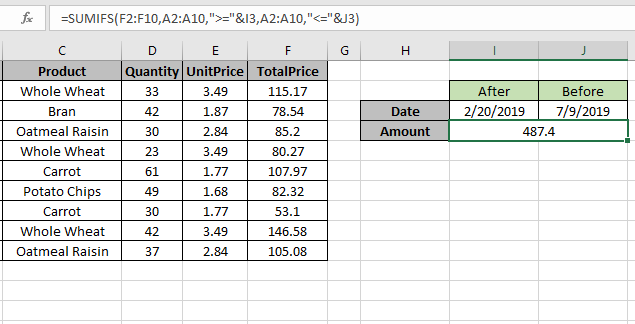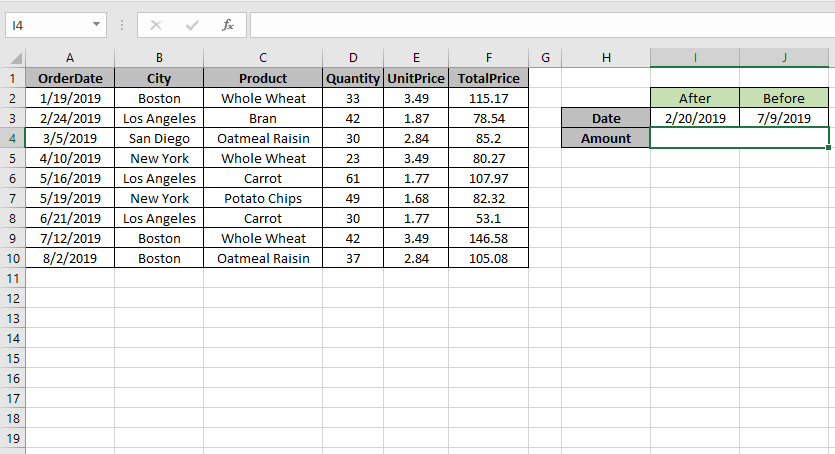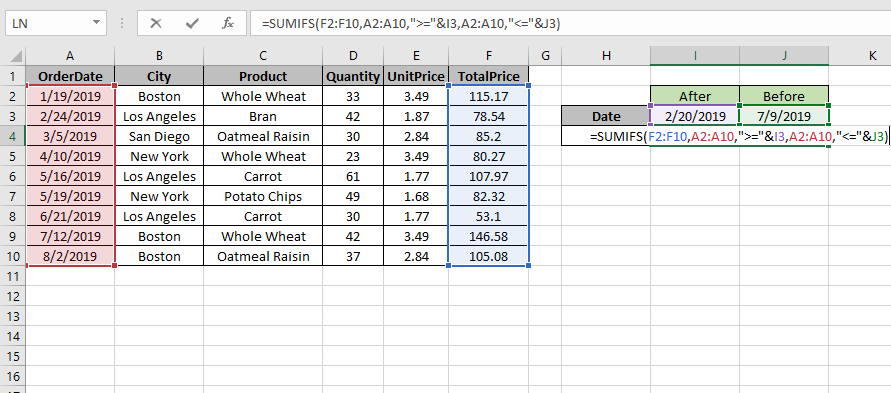# How to SUM if with comparison operator in ExcelIn this article, we will learn How to SUM if with multiple comparison operators in Excel.

SUM values with less than, greater than or equal to given value

Sometimes we need to find the sum if only the value which lay between the required dates or say

we need to add values where the data date of the corresponding values is between the two required dates. For example finding the sales amount in a given month, week, year or any period date gap. Let's understand the formula syntax and an example to illustrate the formula usage.

Operators like equals to ( = ), less than ( < ), less than equal to ( <= ), greater than ( > ), greater than equal to ( >= ) or not equals to ( <> ) can be performed within a formula applied, with numbers only.

SUM if between dates formula in Excel

SUMIFS function returns the sum of range if date is between the

Formula Syntax:

 =SUMIFS(Sum_range , range ,">=" & date1, range, "<=" & date2)

Sum_range : range where sum is required

range : Set of dates

& : operator used to concatenate other operators.

Note: use quote ( " ) marks to provide the type of operator between as shown in syntax or example.

Example :

All of these might be confusing to understand. Let's understand how to use the function using an example. Here we need to find the TotalPrice sum if the date is between 2/20/2019 (after) & 7/9/2019 (before).So we will use the formula to get the amount

 =SUMIFS (F2:F10 , A2:A10 , ">=" & I3, A2:A10 , "<=" & J3)

F2:F10 : Sum_range

A2:A10 : range where condition is applied

">=" & I3 : greater than date value in I3(2/20/2019).

"<=" & J3 : less than date value in J3.(7/9/2019).Use the formula as stated above and click Enter.As you can see the formula returns 487.4, the Totalprice between dates.

For customization just change the dates in I3 & J3 cell and get the results with the formula.

Use the today function, if the end date is required as the last date. You can also use the formula for multiple criteria like date greater than given date (first criteria) and sales range (second criteria) and goes on till the criterias are finished. All these criteria is applied as AND operator (all criterias are matched) not the OR operator (only if one of the criteria matches.

Here are all the observational notes using the formula in Excel
Notes :

1. The formula only works with only to sum up the exact match.
2. Use date references carefully before use. Use the DATE function if the dates are fixed.
3. The function considers non-numeric values as 0s.
4. The function considers the logic value TRUE as 1 and False as 0.
5. The argument array must be the same size else the function returns an error.
6. The function returns the value corresponding to the TRUE values in the returned array after taking individual products in the corresponding array.
7. Operators like equals to ( = ), less than ( < ), less than equal to ( <= ), greater than ( > ), greater than equal to ( >= ) or not equals to ( <> ) can be performed within a formula applied, with numbers only.

Hope this article about How to SUM with multiple comparison operators in Excel is explanatory. Find more articles on summing values and related Excel formulas here. If you liked our blogs, share it with your friends on Facebook. And also you can follow us on Twitter and Facebook. We would love to hear from you, do let us know how we can improve, complement or innovate our work and make it better for you. Write to us at info@exceltip.com.

Related Articles :

Sum by OFFSET groups in Rows and Columns : The OFFSET function can be used to sum group of cells dynamically. These groups can be anywhere in the sheet.

SUM if date is between : Returns the SUM of values between given dates or period in excel.

Sum if date is greater than given date: Returns the SUM of values after the given date or period in excel.

2 Ways to Sum by Month in Excel: Returns the SUM of values within a given specific month in excel.

How to Sum Multiple Columns with Condition: Returns the SUM of values across multiple columns having condition in excel

Use INDEX and MATCH to Lookup Value : The INDEX-MATCH formula is used to lookup dynamically and precisely a value in a given table.  This is an alternative to the VLOOKUP function and it overcomes the shortcomings of the VLOOKUP function.

How to use wildcards in excel : Count cells matching phrases using the wildcards in excel.

Count Cells that contain specific text: A simple COUNTIF function will do the magic. To count the number of multiple cells that contain a given string we use the wildcard operator with the COUNTIF function.

Terms and Conditions of use

The applications/code on this site are distributed as is and without warranties or liability. In no event shall the owner of the copyrights, or the authors of the applications/code be liable for any loss of profit, any problems or any damage resulting from the use or evaluation of the applications/code.